# A step in an equation simplification I don't understand how it is possible

#### dcbingaman

Joined Jun 30, 2021
817
I have the following from another source in an article I was reading: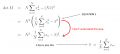Can anyone show the math on how to get from the second step to the third step as shown, Red arrow shows the step I am not understanding.

I get a different result when simplifying (if you want to call this simplifying)>>>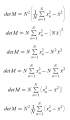Last edited:

#### xox

Joined Sep 8, 2017
795
I have the following from another source in an article I was reading:
View attachment 281475
Can anyone show the math on how to get from the second step to the third step as shown, Red arrow shows the step I am not understanding.
Not exactly sure, but it does have something to do with the fact that x-bar is the average/mean of the dataset. (Because generally speaking, replacing it with any thing else would not yield the same equivalence.) Suffice it to say that for all N > 1, det M equals N^2 times the mean squared error of x.

#### xox

Joined Sep 8, 2017
795
I get a different result when simplifying (if you want to call this simplifying)>>>

View attachment 281476
OK, so that gets you to equation #2. But again, the derivation where you go from that to equation #3 is going to require something other than simple algebraic rearrangement (so number theory or the like). Again, if you replace x-bar with some arbitrary constant for example, the third equation does not necessarily apply.

Ah, here we go, from the Wikipedia article on the arithmetic mean:

The mean is the only single number for which the residuals (deviations from the estimate) sum to zero.
That appears to be the property which allows for the sort of derivation you saw from #2 to #3.

Last edited:
•dcbingaman

#### dcbingaman

Joined Jun 30, 2021
817
OK, so that gets you to equation #2. But again, the derivation where you go from that to equation #3 is going to require something other than simple algebraic rearrangement (so number theory or the like). Again, if you replace x-bar with some arbitrary constant for example, the third equation does not necessarily apply.
I take it you are referring to this: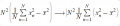That is what I get, but not what the article gets?

#### dcbingaman

Joined Jun 30, 2021
817
OK, so that gets you to equation #2. But again, the derivation where you go from that to equation #3 is going to require something other than simple algebraic rearrangement (so number theory or the like). Again, if you replace x-bar with some arbitrary constant for example, the third equation does not necessarily apply.

Ah, here we go, from the Wikipedia article on the arithmetic mean:

That appears to be the property which allows for the sort of derivation you saw from #2 to #3.
I agree it would not apply. At this point I am wondering if it is not simply wrong?

#### dcbingaman

Joined Jun 30, 2021
817
I agree it would not apply. At this point I am wondering if it is not simply wrong?
I agree it would not apply. At this point I am wondering if it is not simply wrong?

#### dcbingaman

Joined Jun 30, 2021
817
OK, so that gets you to equation #2. But again, the derivation where you go from that to equation #3 is going to require something other than simple algebraic rearrangement (so number theory or the like). Again, if you replace x-bar with some arbitrary constant for example, the third equation does not necessarily apply.

Ah, here we go, from the Wikipedia article on the arithmetic mean:

That appears to be the property which allows for the sort of derivation you saw from #2 to #3.
Could if have something to do with the fact that if you take the mean and multiply it by N you get exactly the same sum as the sum off all xn samples?

#### xox

Joined Sep 8, 2017
795
I agree it would not apply. At this point I am wondering if it is not simply wrong?
No, it is indeed correct, but only because x-bar is the arithmetic mean. Consider the simple fact that, in general (x^2 - y^2) != (x - y)^2. For example, (6^2 - 5^2) = (36 - 25) = 11, but (6 - 5)^2 = 1. So the only way for those two summations to be equivalent would be "some special property" of x-bar.

•dcbingaman

#### dcbingaman

Joined Jun 30, 2021
817
OK, so that gets you to equation #2. But again, the derivation where you go from that to equation #3 is going to require something other than simple algebraic rearrangement (so number theory or the like). Again, if you replace x-bar with some arbitrary constant for example, the third equation does not necessarily apply.

Ah, here we go, from the Wikipedia article on the arithmetic mean:

That appears to be the property which allows for the sort of derivation you saw from #2 to #3.
oh, I found the link, nice link. Pretty involved, I may have to study that for a while.

#### dcbingaman

Joined Jun 30, 2021
817
No, it is indeed correct, but only because x-bar is the arithmetic mean. Consider the simple fact that, in general (x^2 - y^2) != (x - y)^2. For example, (6^2 - 5^2) = (36 - 25) = 11, but (6 - 5)^2 = 1. So the only way for those two summations to be equivalent would be "some special property" of x-bar.
Absolutely, when we foil it it is even more obvious (like the examples with the values you showed):

(x-y)^2=x^2-2xy+y^2

maybe it has something to do with a difference of squares:
x^2-y^2=(x+y)(x-y)?

#### xox

Joined Sep 8, 2017
795
I was just linking the Wikipedia article here.

Could if have something to do with the fact that if you take the mean and multiply it by N you get exactly the same sum as the sum off all xn samples?
No, because even without the terms on the left, the two summations are identical. For example, set x = {5, 13, 30}, so x-bar = 16. And indeed, (5^2 - 16^2) + (13^2 - 16^2) + (30^2 - 16^2) = -231 - 87 + 644 = (5 - 16)^2 + (13 - 16)^2 + (30 - 16)^2 = 326. But now replace x-bar with something else, say 3. Now we have (5^2 - 3^2) + (13^2 - 3^2) + (30^2 - 3^2) = 1067 and (5 - 3)^2 + (13 - 3)^2 + (30 - 3)^2 = 814. And clearly, 1067 != 814.

•dcbingaman

#### dcbingaman

Joined Jun 30, 2021
817
No, it is indeed correct, but only because x-bar is the arithmetic mean. Consider the simple fact that, in general (x^2 - y^2) != (x - y)^2. For example, (6^2 - 5^2) = (36 - 25) = 11, but (6 - 5)^2 = 1. So the only way for those two summations to be equivalent would be "some special property" of x-bar.
It might be correct but how do you go about proving it? I mean we should be able to just replace x bar with its equivalence: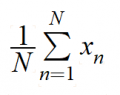And thus prove it, but no matter how much manipulating I do, I get nothing close to that solution.

#### dcbingaman

Joined Jun 30, 2021
817
I was just linking the Wikipedia article here.

No, because even without the terms on the left, the two summations are identical. For example, set x = {5, 13, 30}, so x-bar = 16. And indeed, (5^2 - 16^2) + (13^2 - 16^2) + (30^2 - 16^2) = -231 - 87 + 644 = (5 - 16)^2 + (13 - 16)^2 + (30 - 16)^2 = 326. But now replace x-bar with something else, say 3. Now we have (5^2 - 3^2) + (13^2 - 3^2) + (30^2 - 3^2) = 1067 and (5 - 3)^2 + (13 - 3)^2 + (30 - 3)^2 = 814. And clearly, 1067 != 814.
Indeed that does show they are the same, but how do we prove it algebraically?

#### xox

Joined Sep 8, 2017
795
Indeed that does show they are the same, but how do we prove it algebraically?
I don't think that you can. Well, you would be delving more into the realm of some much deeper maths there anyway.

It is an interesting question though, I will definitely ask around of my more mathematically-literate colleagues to see if there is a simple analytical construct that could be used to prove the equivalence.

•dcbingaman

#### xox

Joined Sep 8, 2017
795
I take it you are referring to this:

View attachment 281484
That is what I get, but not what the article gets?
Sorry, not sure which article you are referring to. In any case, AFAICT the parenthesis are superfluous. Both are equivalent expressions.

#### dcbingaman

Joined Jun 30, 2021
817
Sorry, not sure which article you are referring to. In any case, AFAICT the parenthesis are superfluous. Both are equivalent expressions.
true, my fault, I was thinking the summation only applied to the first term and the second term was subtracted from the summation but that is not correct. It always applies to the entire expression and parenthesis are needed if not. In that case, I may look at it again tomorrow and see if I can find the proof, late here and getting tired.

#### WBahn

Joined Mar 31, 2012
27,942
I'm not too sure what the controversy is all about.

$$N \sum_{n=1}^{N} x^2_n \; - \left(N\bar{x}\right)^2$$

There doesn't appear to be any issue with rewriting this as

$$N^2 \left( \frac{1}{N} \left( \sum_{n=1}^{N} x^2_n \right) \; - \bar{x}^2 \right)$$

I'm putting in an extra parens to make clear what is in the summation and what is not.

This can then be written as

$$N^2 \left( \frac{1}{N} \left(\sum_{n=1}^{N} x^2_n \right) \; - \; 2\bar{x}^2 \; + \; \bar{x}^2 \right)$$

$$N^2 \left( \frac{1}{N} \left(\sum_{n=1}^{N} x^2_n \right) \; - \; 2 \bar{x} \bar{x} \; + \; \bar{x}^2 \right)$$

$$N^2 \left( \frac{1}{N} \left(\sum_{n=1}^{N} x^2_n \right) \; - \; 2 \bar{x} \bar{x} \; + \; \frac{N}{N}\bar{x}^2 \right)$$

There doesn't appear to be any issue with

$$\bar{x} \; = \; \frac{1}{N} \sum_{n=1}^{N}x_n$$

and there shouldn't be a problem with

$$N \; = \; \frac{1}{N} \left( \sum_{n=1}^{N}1 \right)$$

So that gets us to

$$N^2 \left( \frac{1}{N} \left(\sum_{n=1}^{N} x^2_n \right) \; - \; 2 \bar{x} \frac{1}{N} \left( \sum_{n=1}^{N}x_n \right) \; + \; \frac{\left( \sum_{n=1}^{N}1 \right)}{N}\bar{x}^2 \right)$$

We can now factor out the 1/N from the last two terms

$$N^2 \left( \frac{1}{N} \left[ \left(\sum_{n=1}^{N} x^2_n \right) \; - \; 2 \bar{x} \left( \sum_{n=1}^{N}x_n \right) \; + \; \left( \sum_{n=1}^{N}1 \right) \bar{x}^2 \right] \right)$$

Now we can take constants into the summations

$$N^2 \left( \frac{1}{N} \left[ \left(\sum_{n=1}^{N} x^2_n \right) \; - \; \left( \sum_{n=1}^{N} 2 \bar{x} x_n\right) \; + \; \left( \sum_{n=1}^{N} \bar{x}^2 \right) \right] \right)$$

Since the summations are all over the same limits, they can be combined:

$$N^2 \left( \frac{1}{N} \left[ \sum_{n=1}^{N} \left( x^2_n \; - \; 2 \bar{x} x_n \; + \; \bar{x}^2 \right) \right] \right)$$

The summand now reduces to

$$N^2 \left( \frac{1}{N} \left[ \sum_{n=1}^{N} \left( x_n \; - \; \bar{x} \right)^2 \right] \right)$$

Which, after removing some unnecessary parens, agrees with their result

$$N^2 \cdot \frac{1}{N} \sum_{n=1}^{N} \left( x_n \; - \; \bar{x} \right)^2$$

•dcbingaman and xox

#### MrSalts

Joined Apr 2, 2020
2,628
I'm not too sure what the controversy is all about.

$$N \sum_{n=1}^{N} x^2_n \; - \left(N\bar{x}\right)^2$$

There doesn't appear to be any issue with rewriting this as

$$N^2 \left( \frac{1}{N} \left( \sum_{n=1}^{N} x^2_n \right) \; - \bar{x}^2 \right)$$

I'm putting in an extra parens to make clear what is in the summation and what is not.

This can then be written as

$$N^2 \left( \frac{1}{N} \left(\sum_{n=1}^{N} x^2_n \right) \; - \; 2\bar{x}^2 \; + \; \bar{x}^2 \right)$$

$$N^2 \left( \frac{1}{N} \left(\sum_{n=1}^{N} x^2_n \right) \; - \; 2 \bar{x} \bar{x} \; + \; \bar{x}^2 \right)$$

$$N^2 \left( \frac{1}{N} \left(\sum_{n=1}^{N} x^2_n \right) \; - \; 2 \bar{x} \bar{x} \; + \; \frac{N}{N}\bar{x}^2 \right)$$

There doesn't appear to be any issue with

$$\bar{x} \; = \; \frac{1}{N} \sum_{n=1}^{N}x_n$$

and there shouldn't be a problem with

$$N \; = \; \frac{1}{N} \left( \sum_{n=1}^{N}1 \right)$$

So that gets us to

$$N^2 \left( \frac{1}{N} \left(\sum_{n=1}^{N} x^2_n \right) \; - \; 2 \bar{x} \frac{1}{N} \left( \sum_{n=1}^{N}x_n \right) \; + \; \frac{\left( \sum_{n=1}^{N}1 \right)}{N}\bar{x}^2 \right)$$

We can now factor out the 1/N from the last two terms

$$N^2 \left( \frac{1}{N} \left[ \left(\sum_{n=1}^{N} x^2_n \right) \; - \; 2 \bar{x} \left( \sum_{n=1}^{N}x_n \right) \; + \; \left( \sum_{n=1}^{N}1 \right) \bar{x}^2 \right] \right)$$

Now we can take constants into the summations

$$N^2 \left( \frac{1}{N} \left[ \left(\sum_{n=1}^{N} x^2_n \right) \; - \; \left( \sum_{n=1}^{N} 2 \bar{x} x_n\right) \; + \; \left( \sum_{n=1}^{N} \bar{x}^2 \right) \right] \right)$$

Since the summations are all over the same limits, they can be combined:

$$N^2 \left( \frac{1}{N} \left[ \sum_{n=1}^{N} \left( x^2_n \; - \; 2 \bar{x} x_n \; + \; \bar{x}^2 \right) \right] \right)$$

The summand now reduces to

$$N^2 \left( \frac{1}{N} \left[ \sum_{n=1}^{N} \left( x_n \; - \; \bar{x} \right)^2 \right] \right)$$

Which, after removing some unnecessary parens, agrees with their result

$$N^2 \cdot \frac{1}{N} \sum_{n=1}^{N} \left( x_n \; - \; \bar{x} \right)^2$$
Nice job but I don't see the OP's question answered. In post #1 he only asked how this step works because it doesn't make sense to me either...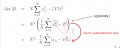#### WBahn

Joined Mar 31, 2012
27,942
Nice job but I don't see the OP's question answered. In post #1 he only asked how this step works because it doesn't make sense to me either...
How is it not answered? I walked from the second equation to the third equation step by step in excrutiating detail. Please indicate which step of that derivation is not clear and I will try to explain that step more carefully.

Last edited:

#### WBahn

Joined Mar 31, 2012
27,942
It's often easiest to figure out the steps by working backwards:

$$N^2 \cdot \frac{1}{N} \sum_{n=1}^{N} \left( x_n \; - \; \bar{x} \right)^2$$

$$N^2 \cdot \frac{1}{N} \sum_{n=1}^{N} \left( x_n^2 \; - \; 2 x_n \bar{x} \; + \; \bar{x}^2 \right)$$

$$N^2 \cdot \frac{1}{N} \left[ \sum_{n=1}^{N} \left( x_n^2 \right) \; - \; \sum_{n=1}^{N} \left( 2 x_n \bar{x} \right) \; + \; \sum_{n=1}^{N} \left( \bar{x}^2 \right) \right]$$

$$N^2 \left( \frac{1}{N} \left[ \sum_{n=1}^{N} \left( x_n^2 \right) \; - \; \sum_{n=1}^{N} \left( 2 x_n \bar{x} \right) \; + \; \sum_{n=1}^{N} \left( \bar{x}^2 \right) \right] \right)$$

$$N^2 \left( \left( \frac{1}{N} \sum_{n=1}^{N} x_n^2 \right) \; - \; \left( 2\bar{x} \frac{\sum_{n=1}^{N} }{N} \right) \; + \; \left( \frac{\bar{x}^2}{N}\sum_{n=1}^{N}1 \right) \right)$$

$$N^2 \left( \left( \frac{1}{N} \sum_{n=1}^{N} x_n^2 \right) \; - \; \left( 2\bar{x} \bar{x} \right) \; + \; \left( \frac{\bar{x}^2}{N}N \right) \right)$$

$$N^2 \left( \left( \frac{1}{N} \sum_{n=1}^{N} x_n^2 \right) \; - \; \left( 2\bar{x}^2 \right) \; + \; \left( \bar{x}^2 \right) \right)$$

$$N^2 \left( \left( \frac{1}{N} \sum_{n=1}^{N} x_n^2 \right) \; - \left( \bar{x}^2 \right) \right)$$

$$N^2 \left( \frac{1}{N} \sum_{n=1}^{N} x_n^2 \; - \bar{x}^2 \right)$$

Here is the starting point, now just walk upward to the ending point.

•dcbingaman and MrSalts